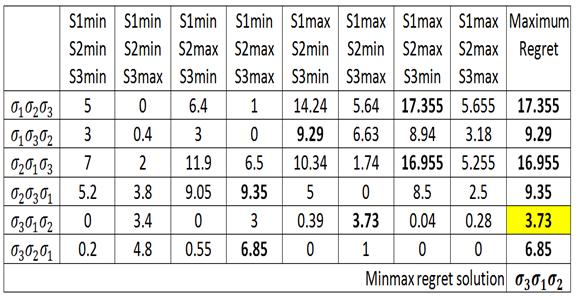Database Query Optimisation Under Uncertainty
Monday, 28 January 2013PhD Student Khaled Alyoubi http://www.dcs.bbk.ac.uk/~khaled Supervisors Dr Peter Wood Dr Sven Helmer Project Details PhD research Keywords Database, Query Optimisation, Minmax Regret Optimisation Database Query Optimisation Under Uncertainty Background Queries in database systems are usually submitted in declarative format. This means that the user formulates the query but does not specify how the database management systems should evaluate the query. The database query optimiser is responsible for deciding how to perform the user's query and should choose the most suitable executable query plan from what is usually a very large number of equivalent plans. The query optimiser uses a cost model to evaluate equivalent plans and choose an efficient one. Cardinality of relations, operator cost per tuple and the selectivity of filtering operators are some of the important parameters that are used by the optimiser in the cost model. While cardinality and operator cost can usually be estimated with reasonable accuracy, selectivity is often the most uncertain and yet one of the most important parameters. Project Aims In our study we assume that the query optimiser is given a set of operators, each with an uncertain selectivity represented as an interval of selectivity values between 0 and 1. An assignment of specific values to all selectivities is called a scenario. The optimiser is not aware of which scenario it will face until run time. The aim is to find the "best" order in which to perform this set of operators. For this purpose we use a Minmax Regret Optimisation Algorithm which tries to minimise the maximum regret of the solution. For a given scenario x, the regret of any plan p is the difference between its cost and the cost of the optimal plan p(opt(x)) (the plan with the smallest cost) for the given scenario x which can be represented as Regret(p,x)=Cost(p,x)-Cost(p(opt(x)),x). Therefore, when the minmax regret solution is confronted with its worst case scenario, it will have the best performance among all other solutions (when confronted with their worst case scenarios). In order to have a better understanding of the problem and to evaluate a number of proposed approaches, we have implemented a simulation tool. Since we suspect that the problem is NP-hard, we are looking for heuristic and approximation algorithms. Example Consider the following operators σ1,σ2 and σ3 with selectivity intervals s1=[.4,.99], s2=[.6,.95] and s3=[.1,.7]. Then the following table shows the regret of all possible scenarios (columns) and all possible execution plans (rows):In each scenario (column) there is a plan with a regret of 0; this is the optimal plan for the scenario. The number in bold text in each row indicates the maximum regret for the plan over all scenarios. The plan which has the smallest maximum regret (highlighted in yellow) is the solution to the minmax regret optimization problem and also corresponds to the optimal execution plan for the operators.